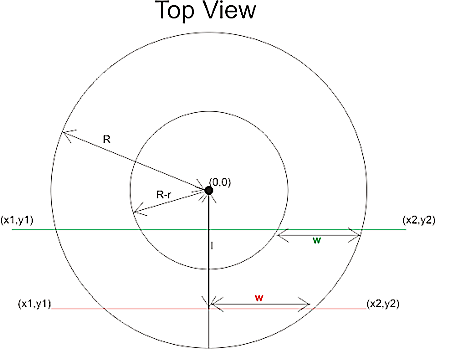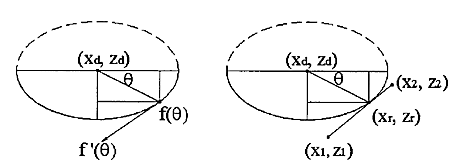# Drop Cutter 3/3: Edge TestThe third and final test in the drop cutter algorithm is to drop the cutter down so it touches an edge. The vertex and facet tests were quite easy, but this one requires a bit of calculations. I'm following Chuang2002.
To simplify things we first translate the tool in the (x, y) plane to (0, 0), and then rotate the edge we are testing against so that it lies along the x-axis and is below the cutter (or on top of the x-axis). The distance from the edge to (0, 0) is l.

Now we imagine a vertical plane through the edge and slice the cutter with that plane. There are two cases, depending on l.If R-r>l we are cutting with a plane (green) that results in an intersection that has two quarter ellipses at the sides and a flat part in the middle. These ellipses have centers with
xd = +/- sqrt( (R-r)^2 - l^2 )

The other case is shown with a red line: if
R-r<=l<R
the intersection of the cutter will be a half ellipse centered at xd=0.
In both cases the curved part of the intersection is described by
f(theta) = (w*cos(theta) , h*cos(theta) ) where 0<theta<pi
h and w, the height and width of the ellipse are given by

• quarter-ellipse case:
• h=r
• w=sqrt(R^r - l^2) - sqrt( (R-r)^2 - l^2 )
• half -ellipse case:
• h=sqrt( r^2 -(l-(R-l))^2 )
• w=sqrt(R^2-l^2)Now that the geometry is clear we can have the edge contact the intersection. That happens at a point where the tangents("slopes") are equal. A tangent vector to the ellipse is given by
f'(theta) = (-w*sin(theta) , -h*cos(theta) )
which can be equated with the slope of the line:
w*sin(theta) / h*cos(theta) = (x1-x2)/(z1-z2)
and we find the angle theta of our CC point:
theta = atan( h*(x1-x2)/w*(z1-z2))
(z1=z2 is a trivial special case not handled here)

The CC point is now given by
xc = xd +/- abs(w*cos(theta))
which should be checked if it lies between x1 and x2. If it does we are contacting the edge, and we can calculate the z-coordinate of the CC point as:
zc = ((xc-x1)/(x2-x1))*(z2-z1) +z1
and finally that leads us to the correct cutter height
ze = zc + abs(h*sin(theta)) - r

Now I need to put all these tests together and find a way of importing STL files into matlab. That way I can begin to test if/how my drop cutter algorithm works!

There's still a lot to do before I have a set of algorithms for basic 3-axis toolpath creation: "push-cutter" a 2D version of drop cutter, 2D line/arc? offsets, zigzag paths, STL-slice with plane, z-buffer simulation of stock material, to name a few things...

## 6 thoughts on “Drop Cutter 3/3: Edge Test”

1.German Camdeveloper says:

I think your algorithm doesn't work
The Geometry of Contact Curve is a cassinische Curve.(no elipse)

2.anders says:

That's an interesting comment, do you have a link to a paper or site which discusses this? some code that solves the problem? a picture?

I will be working with cylindrical and spherical cutters at first but it would be nice to have the harder toroidal cutter working also!

Anders

3.anders says:

I took a look at the papers.

Chuang 2002 clearly talks about ellipses and that's where I took the material for this blog post. That's all wrong, and Yau 2004 seems to have it right.

Apparently the contact point with the cassini oval can't be computed exactly in closed form, so they use a Newton-Rhapson iterative root finding algorithm.

4.oyildirim says:

In half ellipse equations it is written:
h=sqrt( r^2 -(l-(R-l))^2 )
but isn't it h=sqrt( r^2 -(l-(R-r))^2 ) ?

oyildirim

This site uses Akismet to reduce spam. Learn how your comment data is processed.